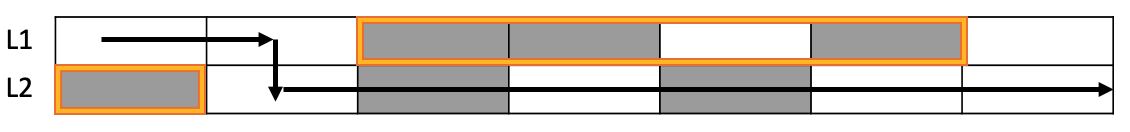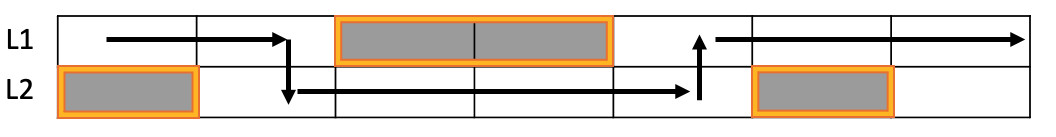# Programming Puzzle: Optimal Pothole Repair

Published:

This post discusses how to efficiently schedule optimal pothole repair!

## Introduction

I encountered a fun programming puzzle recently:

You are given a description of a two-lane road in which two strings, L1 and L2, represent the first and the second lane. Each lane consists of N segments of equal length.

The K-th segment of the first lane is represented by L1[K], and the K-th segment of the second lane is represented by L2[K], where “.” denotes a smooth segment of road, and “x” denotes a segment that contains potholes.

Cars can drive over segments with potholes, but it is uncomfortable for passengers. Therefore, a project to repair as many potholes as possible was submitted. At most one contiguous region of each lane may be repaired at a time. The region is closed to traffic while it is under repair.

How many road segments with potholes can be repaired given that the road must be kept open?

For example, if L1 = “..xx.x.” and L2 = “x.x.x..”, the maximum number of potholes we can repair is 4. See Figure 1 for an explanation.Figure 1: Visualization of the example. Segments without potholes are shown as empty boxes. Segments with potholes are shown as gray boxes. Contiguous regions under repair are highlighted orange. The arrows indicate a path through the road.

## Solution Idea

This problem has two key requirements:

1. Repairs affect a contiguous region. That means that a solution like the one shown in Figure 2 is not allowed.
2. Vehicles must be able to reach the end of the road.Figure 2: L1 = “..xx…” and L2 = “x….x.”. The solution shown here is not allowed, since L2’s repair regions are not contiguous.

There are two important observations about the problem. First, a vehicle must be able to travel the road by changing lanes at most once. I give an argument for this point in the next paragraph. Second, no repair can occur at the segment where the vehicle changes lanes. This is because both lanes must be open for the vehicle to change lanes.

A proof by contradiction shows the vehicle can change lanes at most once in an allowed solution. First, assume without loss in generality that a vehicle starts in L1, and changes lanes twice at segments i and j. A repair must occur in the region [0, i-1] in L2, otherwise the vehicle could have started in L2. A repair must start at segment j in L2, otherwise the vehicle need not change lanes. But the segments [0, i-1] and [j, …] are not contiguous. So, the solution is not allowed. We conclude that a vehicle can change lanes at most once.

Since the vehicle can only change lanes once, we only need to find (1) the segment to change lanes, and (2) the starting lane. Let’s start by characterizing the segment where the vehicle changes lanes. Suppose the vehicle starts in L1. Call the ideal segment to change lanes C. The sum of potholes in L1 in region [C+1, …] and L2 in region […, C-1] is maximal. This is because, since the vehicle doesn’t start in L2, we can repair all segments in L2 until C. The same argument applies to L1 after C.

We can compute C in $O(n)$ time, where n is the number of segments. Maintain two arrays of length n, $avoided_{L1}$ and $avoided_{L2}$. Let $avoided_{L1}[i]$ denote the number of potholes avoided in L1[i+1, …] if the vehicle changes lanes from L1 to L2 at segment i. Similarly, $avoided_{L2}[i]$ denotes the number of potholes avoided in L2[0, i-1] if the vehicles changes lanes from L1 to L2 at segment i. So, $avoided_{L1}$ stores the partial sums of the number of potholes in L1 counting from the end. Meanwhile, $avoided_{L2}$ stores the partial sums of the number of potholes in L2 counting from the start. Computing C is simple: $C = \underset{0 \leq c < n}{\text{argmax}}(avoided_{L1}[c] + avoided_{L2}[c]).$

Finding the starting lane $L$ is also easy. Let $F(A)$ denote the value of $C$ for a vehicle that starts in lane $A$. Then, $L = \underset{l \in \left\{ L1,~ L2 \right\} }{\text{argmax}}(F(l))$.

### Asymptotic Analysis

This solution has a runtime of $O(n)$, since computing $C$ takes $O(n)$ time. Memory usage is $O(n)$, since we create the extra arrays $avoided_{L1}$ and $avoided_{L2}$ to store partial sums.

## Python Implementation

from typing import List

class PotholeState(enum.Enum):
POTHOLE = 1
CLEAN = 2

_STR_TO_STATE = {
'.': PotholeState.CLEAN,
'x': PotholeState.POTHOLE,
}

def read_lanes(l1: str, l2: str) -> List[List[PotholeState]]:
return [[s1, s2] for s1, s2
in zip(
[_STR_TO_STATE[chr] for chr in l1],
[_STR_TO_STATE[chr] for chr in l2],
)]

def _max_repairable_helper(l1: List[PotholeState], l2: List[PotholeState]) -> int:
l1_avoided_potholes =  * len(l1)
l2_avoided_potholes =  * len(l2)
for i in range(len(l1) - 2, -1, -1):
l1_avoided_potholes[i] = l1_avoided_potholes[i + 1]
if l1[i+1] == PotholeState.POTHOLE:
l1_avoided_potholes[i] += 1
for i in range(1, len(l2)):
l2_avoided_potholes[i] = l2_avoided_potholes[i - 1]
if l2[i - 1] == PotholeState.POTHOLE:
l2_avoided_potholes[i] += 1
return max([l1_avoided_potholes[i] + l2_avoided_potholes[i] for i in range(len(l1))])

def max_repairable_segments(road: List[List[PotholeState]]) -> int:
lane1 = [road[i] for i in range(len(road))]
lane2 = [road[i] for i in range(len(road))]
return max(
_max_repairable_helper(lane1, lane2),
_max_repairable_helper(lane2, lane1),
)


## Follow-ups

• Remove the requirement that only one contiguous region per lane can be under repair.
• Find the regions under repair in both lanes in an optimal solution.
• There are two key properties in a solution. Check the implementation with property tests.

Tags: